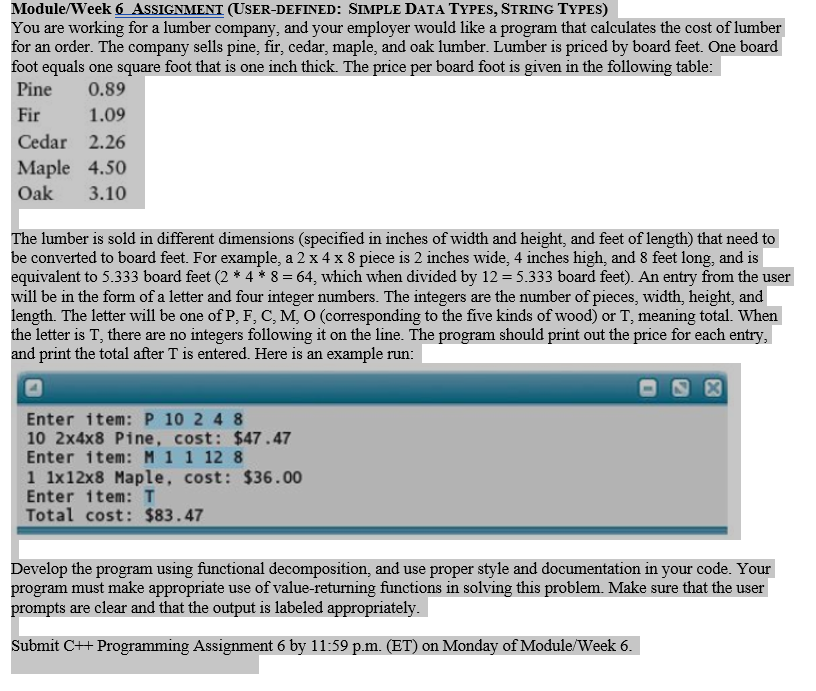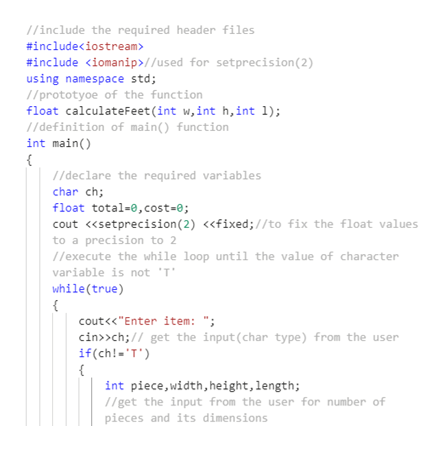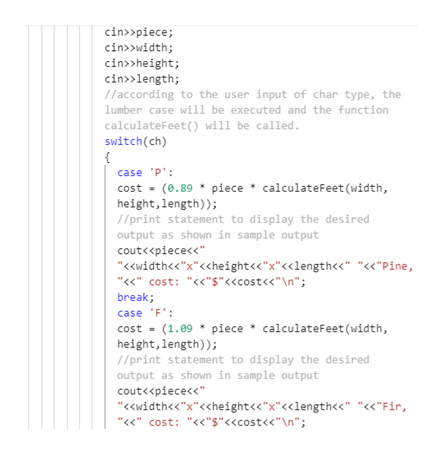# Module/Week 6 ASSIGNMENT (USER-DEFINED: SIMPLE DATA TYPES, STRING TYPES)You are working for a lumber company, and your employer would like a program that calculates the cost of lumberfor an order. The company sells pine, fir, cedar, maple, and oak lumber. Lumber is priced by board feet. One boardfoot equals one square foot that is one inch thick. The price per board foot is given in the following table:Pine0.891.09FirCedar 2.26Maple 4.50Oak3.10The lumber is sold in different dimensions (specified in inches of width and height, and feet of length) that need tobe converted to board feet. For example, a 2 x 4 x 8 piece is 2 inches wide, 4 inches high, and 8 feet long, and isequivalent to 5.333 board feet (2 *4* 8 = 64, which when divided by 12 = 5.333 board feet). An entry from the userwill be in the form of a letter and four integer numbers. The integers are the number of pieces, width, height, andlength. The letter will be one of P, F, C, M, O (corresponding to the five kinds of wood) or T, meaning total. Whenthe letter is T, there are no integers following it on the line. The program should print out the price for each entry,and print the total after T is entered. Here is an example run:Enter item: P 10 2 4 810 2x4x8 Pine, cost: \$47.47Enter item: M 1 1 12 81 1x12x8 Maple, cost: \$36.00Enter 1tem: TTotal cost: \$83.47Develop the program using functional decomposition, and use proper style and documentation in your code. Yourprogram must make appropriate use of value-returning functions in solving this problem. Make sure that the userprompts are clear and that the output is labeled appropriatelySubmit C++ Programming Assignment 6 by 11:59 p.m. (ET) on Monday of Module/Week 6

Question
24 views

Module/Week 6 Assignment (User-defined: Simple Data Types, String Types)

You are working for a lumber company, and your employer would like a program that calculates the cost of lumber for an order. The company sells pine, fir, cedar, maple, and oak lumber. Lumber is priced by board feet. One board foot equals one square foot that is one inch thick. The price per board foot is given in the following table:

The lumber is sold in different dimensions (specified in inches of width and height, and feet of length) that need to be converted to board feet. For example, a 2 x 4 x 8 piece is 2 inches wide, 4 inches high, and 8 feet long, and is equivalent to 5.333 board feet (2 * 4 * 8 = 64, which when divided by 12 = 5.333 board feet). An entry from the user will be in the form of a letter and four integer numbers. The integers are the number of pieces, width, height, and length. The letter will be one of P, F, C, M, O (corresponding to the five kinds of wood) or T, meaning total. When the letter is T, there are no integers following it on the line. The program should print out the price for each entry, and print the total after T is entered. Here is an example run:

Develop the program using functional decomposition, and use proper style and documentation in your code. Your program must make appropriate use of value-returning functions in solving this problem. Make sure that the user prompts are clear and that the output is labeled appropriately.

Submit C++ Programming Assignment 6 by 11:59 p.m. (ET) on Monday of Module/Week 6.help_outlineImage TranscriptioncloseModule/Week 6 ASSIGNMENT (USER-DEFINED: SIMPLE DATA TYPES, STRING TYPES) You are working for a lumber company, and your employer would like a program that calculates the cost of lumber for an order. The company sells pine, fir, cedar, maple, and oak lumber. Lumber is priced by board feet. One board foot equals one square foot that is one inch thick. The price per board foot is given in the following table: Pine 0.89 1.09 Fir Cedar 2.26 Maple 4.50 Oak 3.10 The lumber is sold in different dimensions (specified in inches of width and height, and feet of length) that need to be converted to board feet. For example, a 2 x 4 x 8 piece is 2 inches wide, 4 inches high, and 8 feet long, and is equivalent to 5.333 board feet (2 *4* 8 = 64, which when divided by 12 = 5.333 board feet). An entry from the user will be in the form of a letter and four integer numbers. The integers are the number of pieces, width, height, and length. The letter will be one of P, F, C, M, O (corresponding to the five kinds of wood) or T, meaning total. When the letter is T, there are no integers following it on the line. The program should print out the price for each entry, and print the total after T is entered. Here is an example run: Enter item: P 10 2 4 8 10 2x4x8 Pine, cost: \$47.47 Enter item: M 1 1 12 8 1 1x12x8 Maple, cost: \$36.00 Enter 1tem: T Total cost: \$83.47 Develop the program using functional decomposition, and use proper style and documentation in your code. Your program must make appropriate use of value-returning functions in solving this problem. Make sure that the user prompts are clear and that the output is labeled appropriately Submit C++ Programming Assignment 6 by 11:59 p.m. (ET) on Monday of Module/Week 6 fullscreen
check_circle

Step 1

C++ Source Code

#include<iostream>

#include <iomanip>//used for setprecision(2)

using namespace std;

//prototyoe of the function

float calculateFeet(int w,int h,int l);

//definition of main() function

int main()

{

//declare the required variables

char ch;

float total=0,cost=0;

cout <<setprecision(2) <<fixed;//to fix the float values to a precision to 2

//execute the while loop until the value of character variable is not 'T'

while(true)

{

cout<<"Enter item: ";

cin>>ch;// get the input(char type) from the user

if(ch!='T')

{

int piece,width,height,length;

//get the input from the user for number of pieces and its dimensions

cin>>piece;

cin>>width;

cin>>height;

cin>>length;

//according to the user input of char type, the lumber case will be executed and the function calculateFeet() will be called.

switch(ch)

{

case 'P':

cost = (0.89 * piece * calculateFeet(width,height,length));

//print statement to display the desired output as shown in sample output

cout<<piece<<" "<<width<<"x"<<height<<"x"<<length<<" "<<"Pine,"<<" cost: "<<"\$"<<cost<<" ";

break;

case 'F':

cost = (1.09 * piece * calculateFeet(width,height,length));

//print statement to display the desired output as shown in sample output

cout<<piece<<" "<<width<<"x"<<height<<"x"<<length<<" "<<"Fir,"<<" cost: "<<"\$"<<cost<<" ";

break;

case 'C':

cost = (2.26 * piece * calculateFeet(width,height,length));

//print statement to display the desired output as shown in sample output

cout<<piece<<" "<<width<<"x"<<height<<"x"<<length<<" "<<"Cedar,"<<" cost: "<<"\$"<<cost<<" ";

break;

case 'M':

cost = (4.50 * piece * calculateFeet(width,height,length));

//print statement to display the desired output as shown in sample output

cout<<piece<<" "<<width<<"x"<<height<<"x"<<length<<" "<<"Maple,"<<" cost: "<<"\$"<<cost<<" ";

break;

case 'O':

cost = (3.10 * piece * calculateFeet(width,height,length));

//print statement to display the desired output as shown in sample output

cout<<piece<<" "<<width<<"x"<<height<<"x"<<length<<" "<<"Oak,"<<" cost: "<<"\$"<<cost<<" ";

break;

}

total += cost;//add cost to total

}

//if user input char 'T' then print total cost and exit

else

{

//print statement to display the total cost when the user input char 'T'

cout<<"Total cost: \$"<<total<<" ";

break;

}

}

return 0;

}//end of main() function

//definition of the called function

float calculateFeet(int w,int h,int l)

{

float feet = ((w*h*l)/12.0);

return feet;

}

Step 2

Screenshot 1help_outlineImage Transcriptionclose//include the required header files #include #include //used for setprecision (2) using namespace std /prototyoe of the function float calculateFeet (int w,int h,int 1); //definition of main () function int main) //declare the required variables char ch; oat total-e,cost- ; cout <>ch;// get the input(char type) from the user if(ch!-T') { int piece,width, height, length; //get the input from the user for number of pieces and its dimensions fullscreen
Step 3

Screenshot 2

...help_outlineImage Transcriptionclosecin>piece; cinwidth; cin>>height; cin>length; //according to the user input of char type, the lumber case will be executed and the function calculateFeet() will be called switch(ch) { case P' cost (e.89 piece * calculateFeet (width, height,length)); //print statement to display the desired output as shown in sample output cout<

### Want to see the full answer?

See Solution

#### Want to see this answer and more?

Solutions are written by subject experts who are available 24/7. Questions are typically answered within 1 hour.*

See Solution
*Response times may vary by subject and question.
Tagged in

### Computer Science